# Converting Scientific Notation Scientific Notation is a way

• Slides: 12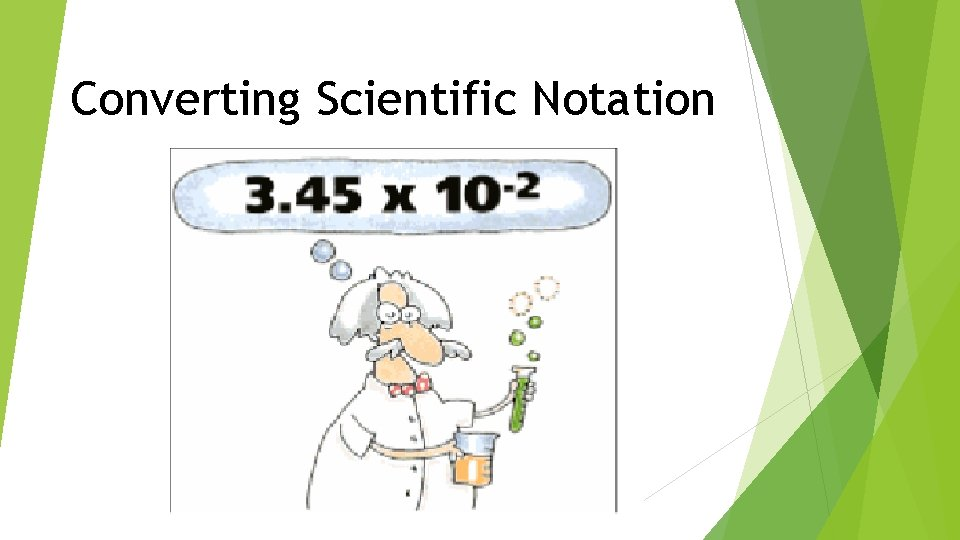Converting Scientific NotationScientific Notation is a way of writing very large or very small numbers that are hard to express in decimal form. A number is written in scientific notation if it is of the form c x 10 n Where c is between 1 and 10 and n is an integer. Reminder: an integer is a positive, negative whole number including zero {…. -3, -2, -1, 0, 1, 2, 3…. }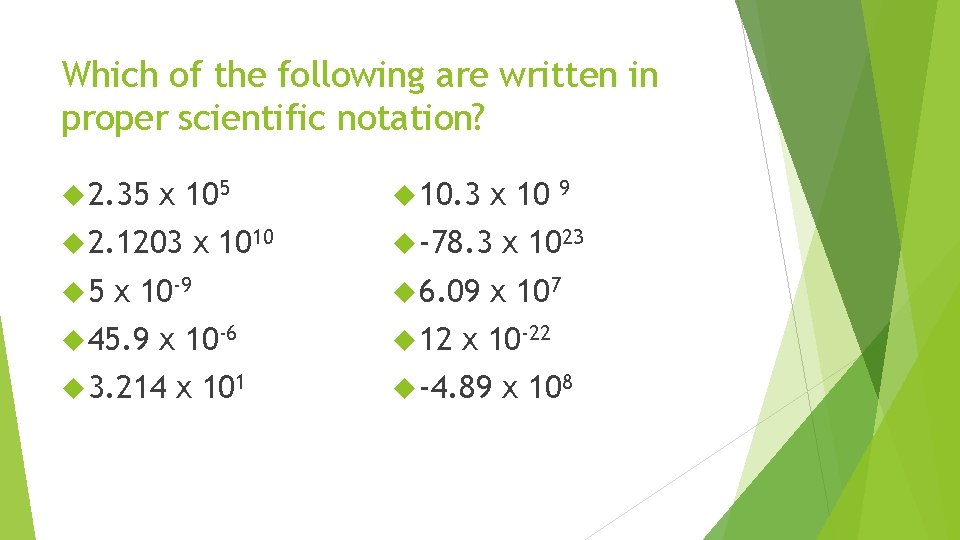Which of the following are written in proper scientific notation? 2. 35 x 105 2. 1203 5 x 1010 x 10 -9 45. 9 x 10 -6 3. 214 x 101 10. 3 x 10 -78. 3 6. 09 12 9 x 1023 x 107 x 10 -22 -4. 89 x 108Scientific Notation is used to write…. REALLY big numbers Decimal Notation 123, 000, 000. 45, 000. 67, 800, 000, 000. Scientific NotationScientific Notation is used to write…. REALLY big numbers Scientific Notation 7. 82 x 10 3 3. 04 x 108 5 x 104 Decimal NotationHere’s an example…. As the planets orbit the sun, the closest Pluto gets to Earth is approximately 2, 700, 000 miles. Write that number in scientific notation.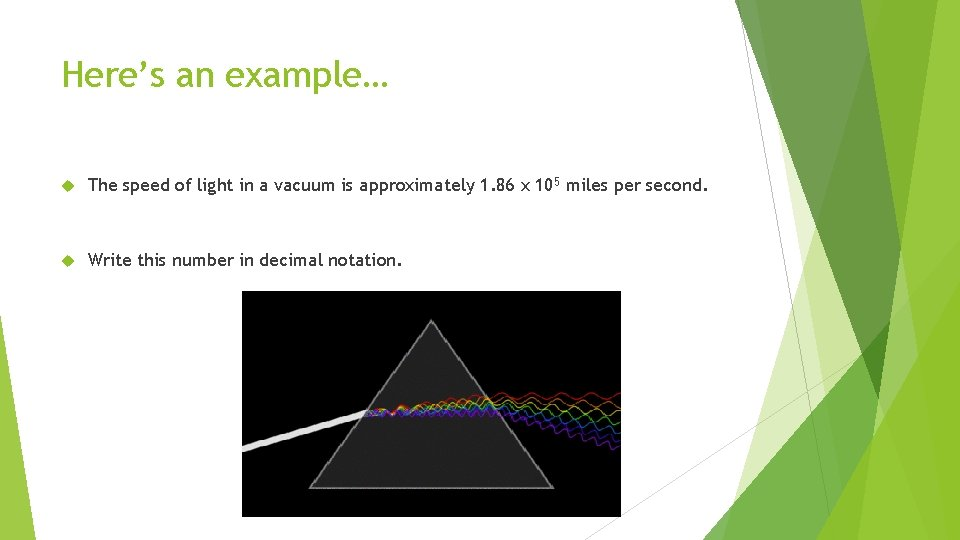Here’s an example… The speed of light in a vacuum is approximately 1. 86 x 105 miles per second. Write this number in decimal notation.Scientific Notation is used to write…. REALLY small numbers Decimal Notation 0. 000000034 0. 000005609 0. 0000064 Scientific Notation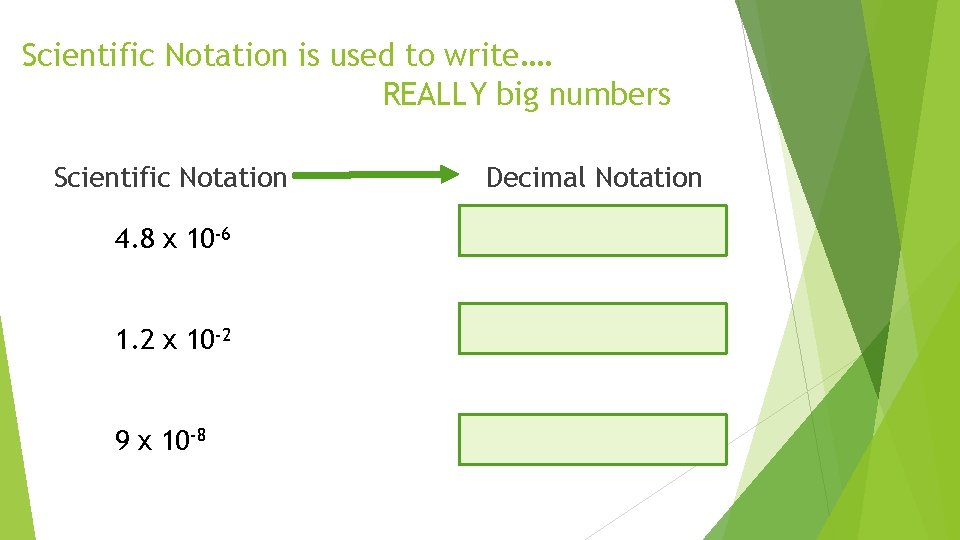Scientific Notation is used to write…. REALLY big numbers Scientific Notation 4. 8 x 10 -6 1. 2 x 10 -2 9 x 10 -8 Decimal NotationHere’s an example… Human fingernails grow at a rate of about 0. 00286 inches per day. Write this number in scientific notation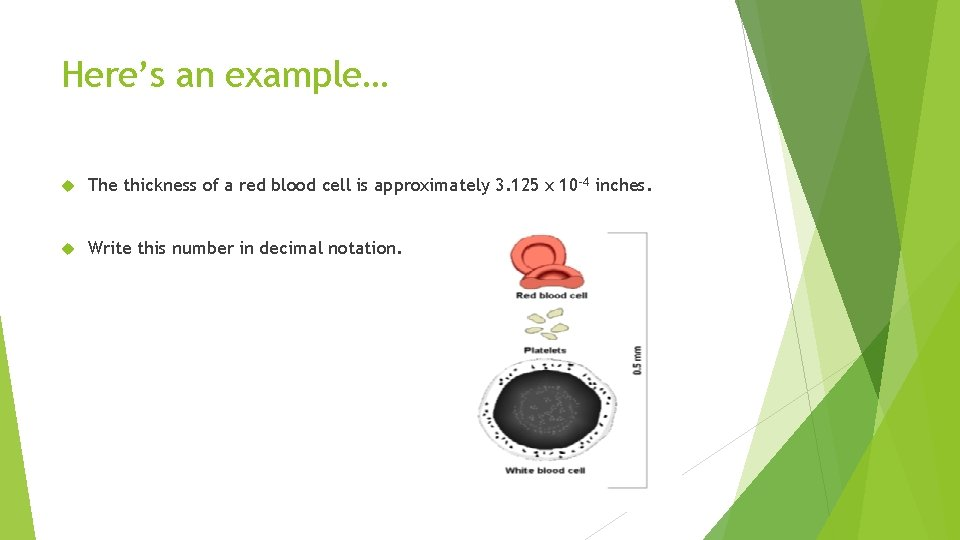Here’s an example… The thickness of a red blood cell is approximately 3. 125 x 10 -4 inches. Write this number in decimal notation.What about the exponents? When do you write a negative exponent when converting to scientific notation? When do you write a positive exponent when converting to scientific notation?